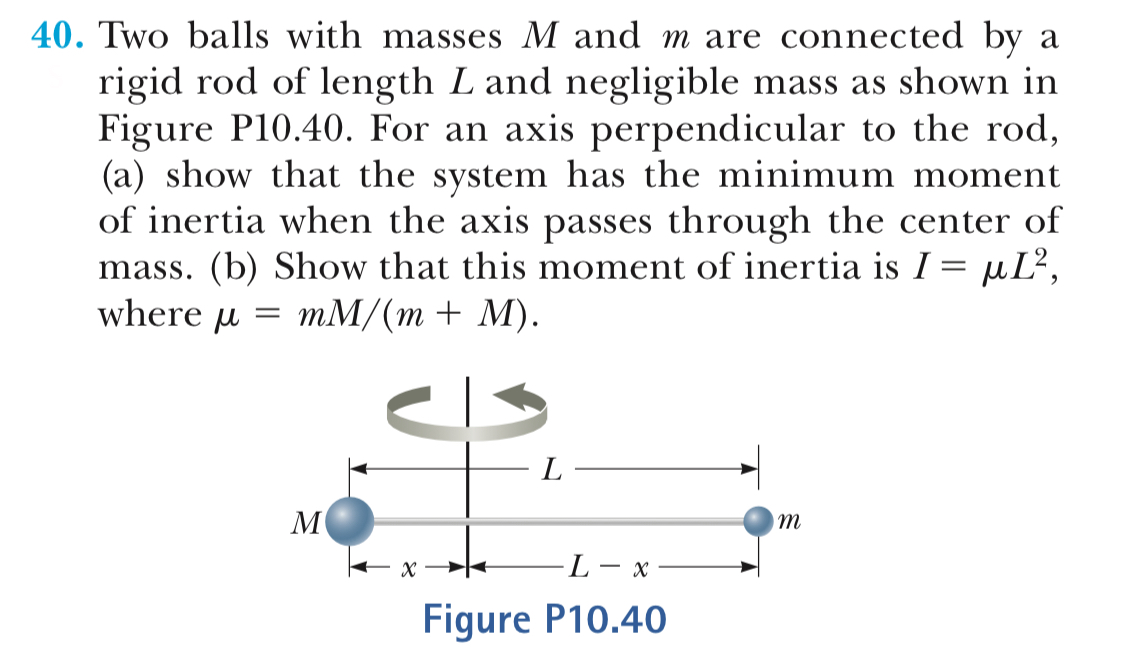# 40. Two balls with masses M and m are connected by rigid rod of length L and negligible mass as shown in Figure P10.40. For an axis perpendicular to the rod (a) show that the system has the minimum moment of inertia when the axis passes through the center of mass. (b) Show that this moment of inertia is Г-, L2 where umM/(m+ M) Figure P10.40

Questionhelp_outlineImage Transcriptionclose40. Two balls with masses M and m are connected by rigid rod of length L and negligible mass as shown in Figure P10.40. For an axis perpendicular to the rod (a) show that the system has the minimum moment of inertia when the axis passes through the center of mass. (b) Show that this moment of inertia is Г-, L2 where umM/(m+ M) Figure P10.40 fullscreen

### Want to see this answer and more?

Step-by-step answers are written by subject experts who are available 24/7. Questions are typically answered in as fast as 30 minutes.*